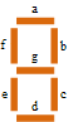TL;DR

On with TASK #2 from The Weekly Challenge #200. Enjoy!

# The challenge

A seven segment display is an electronic component, usually used to display digits. The segments are labeled 'a' through 'g' as shown:The encoding of each digit can thus be represented compactly as a truth table:

my @truth = qw<abcdef bc abdeg abcdg bcfg acdfg a cdefg abc abcdefg abcfg>;


For example, $truth = ‘bc’. The digit 1 would have segments ‘b’ and ‘c’ enabled. Write a program that accepts any decimal number and draws that number as a horizontal sequence of ASCII seven segment displays, similar to the following: ------- ------- ------- | | | | | | | | | | ------- | | | | | | | | | | ------- ------- -------  To qualify as a seven segment display, each segment must be drawn (or not drawn) according to your @truth table. The number "200" was of course chosen to celebrate our 200th week! # The questions Do you know that there is a stray space in the representation of the number 6?!? # The solution Each line of the output depends on either one or two segments. In particular, the a, d, and gdetermine horizontal segments, while the other ones contribute to vertical ones, pair by pair. This is what we will be using in our Perl code, then: #!/usr/bin/env perl use v5.24; use warnings; use experimental 'signatures'; no warnings 'experimental::signatures'; say render_seven_segment(shift // 200); sub render_seven_segment ($number) {
state $truth = [ map { +{ map {$_ => 1 } split m{}mxs, $_ } } qw<abcdef bc abdeg abcdg bcfg acdfg acdefg abc abcdefg abcfg> ]; state$h_line = sub ($letter, @digits) { state$segment = [ ' ' x 7, ' ' . ('-' x 5) . ' ' ];
join ' ',
map { $segment->[$truth->[$_]{$letter} ? 1 : 0] } @digits
};
state $v_line = sub ($left, $right, @digits) { state$sep = ' ' x 5;
join ' ', map {
my $digit =$truth->[$_]; join$sep, map { $digit->{$_} ? '|' : ' ' } ($left,$right);
} @digits;
};

my @digits = split m{}mxs, $number; return join "\n",$h_line->('a', @digits),
($v_line->('f', 'b', @digits)) x 2,$h_line->('g', @digits),
($v_line->('e', 'c', @digits)) x 2,$h_line->('d', @digits);

}


It’s easy to translate this into Raku, although there’s probably a more idiomatic way of doing this. Whatever!

#!/usr/bin/env raku
use v6;
sub MAIN ($number = 200) { put render-seven-segment($number) }

sub render-seven-segment ($number) { state @truth = <abcdef bc abdeg abcdg bcfg acdfg acdefg abc abcdefg abcfg> .map: { .comb.map({$_ => 1}).Hash };
sub h_line ($letter, @digits) { state @segment = ' ' x 7, ' ' ~ ('-' x 5) ~ ' '; @digits.map({ @segment[@truth[$_]{$letter} ?? 1 !! 0] }).join(' '); } sub v_line ($left, $right, @digits) { state$sep = ' ' x 5;
@digits.map({
my $d = @truth[$_];
($left,$right).map({$d{$_} ?? '|' !! ' '}).join($sep); }).join(' '); } my @digits =$number.comb;
(
h_line('a', @digits),
v_line('f', 'b', @digits) xx 2,
h_line('g', @digits),
v_line('e', 'c', @digits) xx 2,
h_line('d', @digits),
).flat.join: "\n";
}


Stay safe!

Comments? Octodon, , GitHub, Reddit, or drop me a line!